# Albanese variety

(diff) ← Older revision | Latest revision (diff) | Newer revision → (diff)

An Abelian variety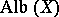canonically attached to an algebraic variety, which is the solution of the following universal problem: There exists a morphismsuch that any morphisminto an Abelian varietyfactors into a product, where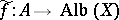(so named in honour of G. Albanese). Ifis a complete non-singular variety over the field of complex numbers, the Albanese variety can be described as follows. Let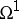be the space of everywhere-regular differential forms of degree 1 on. Each one-dimensional cycleof the topological spacedetermines a linear function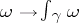on. The image of the mapping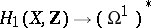thus obtained is a latticein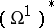, and the quotient space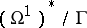coincides with the Albanese variety of. From the algebraic point of view, an Albanese variety may be considered as a method of defining an algebraic structure on some quotient group of the groupof zero-dimensional cycles of degree 0 on. Ifis a non-singular complete algebraic curve, both its Picard variety and its Albanese variety are called its Jacobi variety. If the ground field has characteristic zero, then the equalities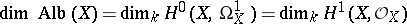are valid. The number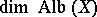is called the irregularity of the variety. If the field has finite characteristic, the inequalities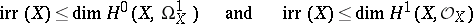hold. If the ground field has positive characteristics it can happen thatThe Albanese variety is dual to the Picard variety.

How to Cite This Entry:
Albanese variety. Encyclopedia of Mathematics. URL: http://encyclopediaofmath.org/index.php?title=Albanese_variety&oldid=18712
This article was adapted from an original article by A.N. Parshin (originator), which appeared in Encyclopedia of Mathematics - ISBN 1402006098. See original article